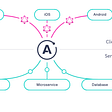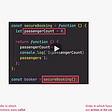# Rest Operator

`function sum(a,b){return a+b;}console.log(sum(4,5))`
`// more parameters than argumentsfunction sum(a,b){return a+b;}console.log(sum(4)) //output: NaN`
`// more arguments than parametersfunction sum(a,b){return a+b;}console.log(sum(4,5,6,7)) // output: 9`
`function sum(…rest){let sum = 0;for(let i =0; i<rest.length; i++){sum += rest[i]}return sum}console.log(sum(4,5,6,7)) // output: 22`
`function sum(a, …rest){let sum = a;for(let i =0; i<rest.length; i++){sum += rest[i]}return sum}console.log(sum(4,5,6,7)) // output: 22`
`function sum(a, …rest, b){ // error : Rest parameter must be last formal parameterlet sum = a;for(let i =0; i<rest.length; i++){sum += rest[i]}return sum}console.log(sum(4,5,6,7))`

`function sum(a, …rest){let sum = a;for(let i =0; i<rest.length; i++){sum += rest[i]}return sum}const arr = [4,5,6,7]console.log(sum(…arr)) // output: 22`
`let arr = [1,2,3]console.log(Math.max(…arr)) //output: 3`
`let arr = [1,2,3]let arr1 = [4,5,6]let newArr = [ …arr, …arr1 , 7]console.log(newArr) //output: [ 1, 2, 3, 4, 5, 6, 7 ]`
`let obj = {a:1, b:2, c:3}let objCopy = { …obj }console.log(objCopy) // output: { a: 1, b: 2, c: 3 }`
`let arr = [1,2,1,4,3,3,1]let newArr = […new Set(arr)]console.log(newArr) // output: [ 1, 2, 4, 3 ]We can also use the in strings.let str= “Hello”console.log([…str]) //output : [ ‘H’, ‘e’, ‘l’, ‘l’, ‘o’ ]`
`console.log([…str]) //output : [ ‘H’, ‘e’, ‘l’, ‘l’, ‘o’ ]`

# Conclusion

--

--

--

## More from Serajur Reza Chowdhury

Software Engineer, curious JavaScript programmer, love to share concepts.

Love podcasts or audiobooks? Learn on the go with our new app.

## Resilient JavaScript with Sanctuary## Seriously Strict Script## Why prefer GraphQL over REST API## Building native apps with JavaScript: Titanium## Random walk and colored dots## Android: Detect when app is in foreground or background## Serajur Reza Chowdhury

Software Engineer, curious JavaScript programmer, love to share concepts.

## JavaScript Some Key Topics## JavaScript - Callbacks, Promises, and Async/Await